Scan QR code or get instant email to install app

Question:

# Which of the following is the solution to the triangle given?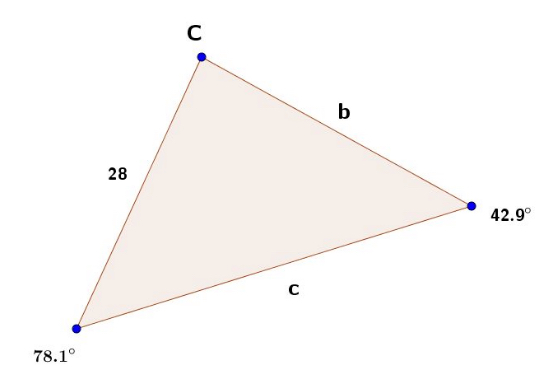A 1212b = 40.2, c = 35.3,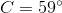explanation

1212Recall that the sum of the interior angles of any triangle equals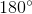. Because 2 angles are given, the third can be found as follows: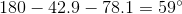To solve for the side lengths, use the law of sines: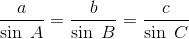.
Solving for b: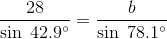which gives
b = 40.2
Performing the same operation to solve for side length c: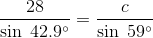which gives
c = 35.3